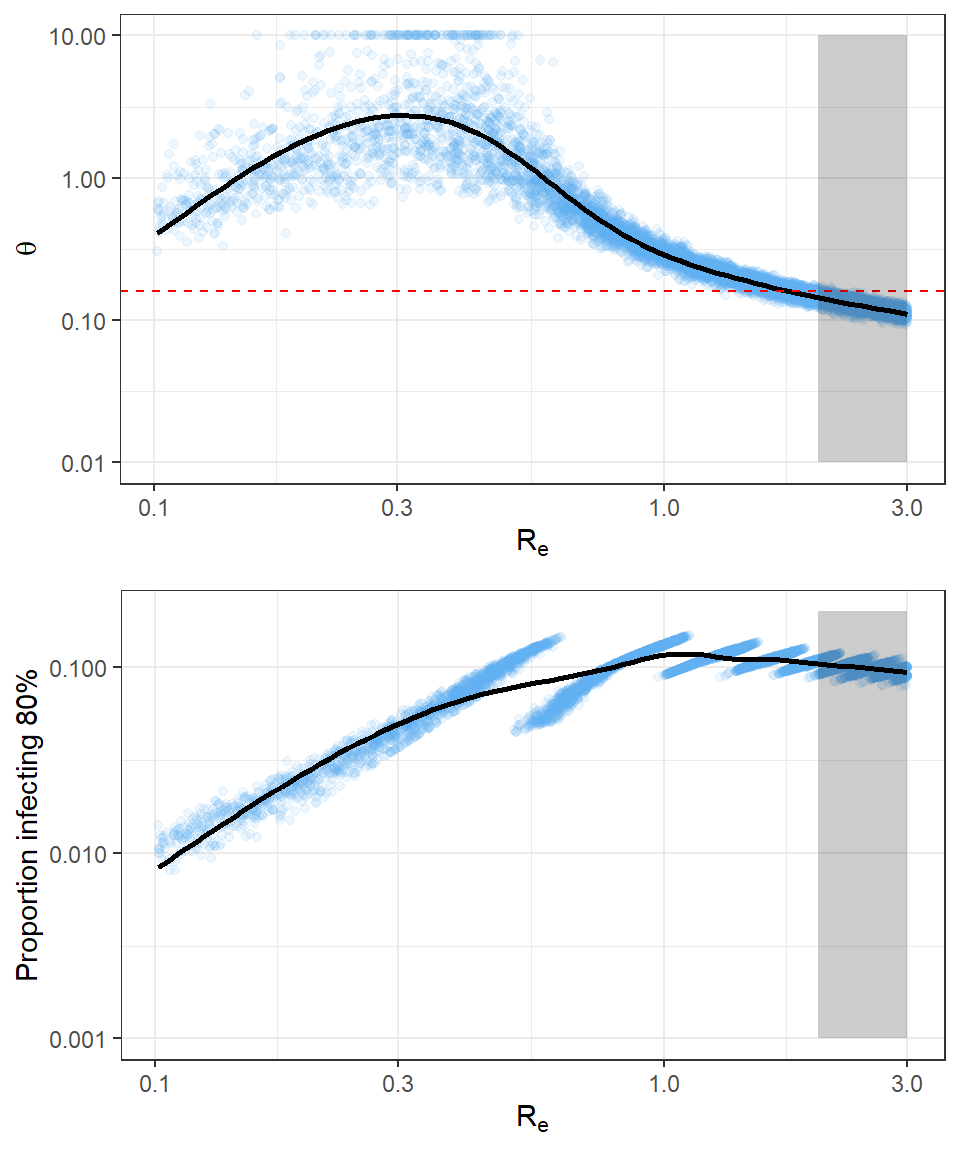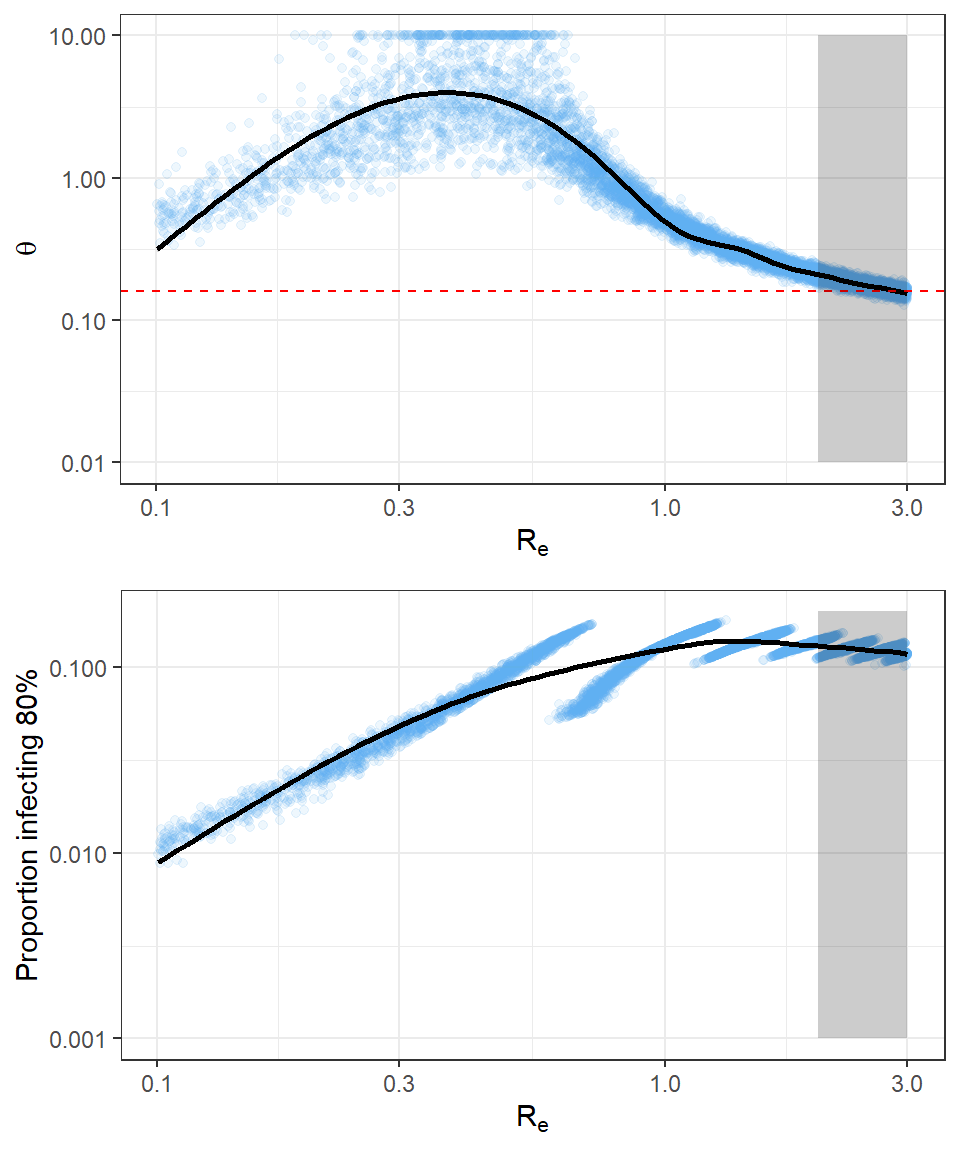NOTE (2020-15-2): Update at bottom based on numbers as of February 15 2020.

Despite introductions of the novel coronavirus into 27 countries, as of February 11, 2020, there has been little documented onward transmission outside of China. At the start of the outbreak, the basic reproductive number, $$R_0$$, was estimated to be somewhere between 2 and 3 in Wuhan. That is, early growth features of the outbreak indicate that, on average, one infected individual infects between 2 and 3 other individuals. However, this same rate of epidemic growth has not been observed outside of China (and perhaps even in other provinces within China) despite numerous introductions.

These apparently contradictory observations can be reconciled in two ways: (A) onward transmission of the virus is less likely outside of China, presumably due to case finding paired with isolation and quarantine, i.e. the effective reproductive number $$R_e$$ is reduced; or (B) transmission of COVID-19 is in general overdispersed, i.e., the majority of transmission is due to a few superspreading events, while the vast majority of infected individuals do not transmit the virus. Perhaps most likely is that we are seeing some combination of these two effects.

By examining the number of introductions that have failed to result in onward transmission, we can get a sense of how extreme each of these effects has to be, and how they might work in combination, to produce the observed results. Following Lloyd-Smith et al., 2005, we assume that the number of secondary cases associated with an infectious individual is drawn from a negative binomial distribution with population mean $$R_e$$ and dispersion parameter $$\theta$$, where this distribution encodes individual characteristics of contact, environment, etc., that might modulate individual onward transmission. We estimate a range of $$R_e$$ and $$\theta$$ values consistent with the observed number of secondary transmission events outside of China to reconcile the seemingly contradictory observations of epidemic growth.

#### Data

We use data from the WHO Situation Report on the number of assumed imported (n=165) and local cases (n=84) in 24 countries to simulate an expected number of secondary infections caused by each known infection within country, assuming a uniform distribution of onward transmission events across all known cases. We repeat this simulation process 10,000 times to model the uncertainty in the data.

nreps = 10000

## Generate datasets from known number of cases (total + assumed local transmission in each country)
sim_data <- function(nreps, ntotal.vec, nlocal.vec){
tmp <- matrix(rep(0, nreps), nrow=1)
for(i in 1:length(ntotal.vec)){
tmp <- rbind(tmp, rmultinom(nreps, nlocal.vec[i], rep(1/ntotal.vec[i], ntotal.vec[i])))
}
return(tmp[-1,])
}

tmp.dat <- sim_data(nreps, country.dat$total.cases, country.dat$cases.outside)

#### Dispersion parameter estimation

We then find the optimal dispersion parameter, $$\theta$$, that best makes each assumed simulated data set consistent with a random value of $$R_e$$ drawn from a plausible range (0.1 - 3). Individual variation in infectiousness implies outbreaks are rarer but more explosive. Interpreting the $$\theta$$ parameter is eased by framing it in terms of the fraction of individuals responsible for 80% of onward transmission (by analogy with the 20/80 rule).

Key functions used in the analysis:

## likelihood function for theta to optimize
to_optim <- function(theta, R0, data) {
-sum(dnbinom(x=data, size=theta,  mu=R0, log = TRUE))
}

## optimization function
do_optim <- function(R0, data) {
return(optimize(to_optim,c(0,5000),R0=R0, data=data)\$minimum)
}

## Calculate proportion to infect some percentage of the population given
## Re and theta.
getPropInfected <- Vectorize(function(Re,theta,target.prop=0.80) {
max.x <- 5000
tmp <- dnbinom(1:max.x, mu=Re, size=theta) * 1:max.x
tmp2 <- rev(cumsum(rev(tmp)))/sum(tmp)
rc<-1-pnbinom(which.min(abs(tmp2-target.prop)), mu=Re, size=theta)
return(rc)

})Figure (top) The optimal $$\theta$$ versus $$R_e$$ over 10,000 simulated data sets and (bottom) the proportion responsible for 80% of onward transmission. Red dashed horizontal line represents the dispersion estimated for SARS in Singapore, and the shaded region represents a rough range of plausible $$R_e$$ for the outbreak of COVID-19 in Wuhan.

We find a range of possible values of over-dispersion consistent with the observed data at $$R_e = 2$$, as well as reduced mean $$R_e$$ values. We estimate that, if $$R_e$$ outside of China were between 2 and 3, as estimated within Wuhan, just 9.9% (range 7.9, 11.9 ) of all infections would be responsible for 80% of onward transmission events. On average, the estimated dispersion parameter $$\theta$$ is less than that estimated for SARS in 2003, indicating that there may be more skew in the distribution of individual $$R_e$$ for COVID-2019 than for SARS. If $$R_e$$ was reduced to just below 1, in the 0.5-0.1 range, then 10.0% (range 4.5, 11.9 ) would be responsible for 80% of onward transmission.

#### Conclusions

This analysis indicates the relative lack of observed onward transmission following introductions of COVID-19 outside of China is consistent with moderate levels of over-dispersion and $$R_e$$ in the range of what has been observed in Wuhan ($$R_e$$ = 2-3). This suggests we should be cautious about assuming the relative lack of COVID-19 transmission outside of China is the result of effective control measures, or some other fundamental difference in COVID-19 transmission outside of Wuhan (and China more broadly).

These results are driven by the near absence of secondary infections emerging from international infections, and there may be a number of onward transmissions that are unobserved, in which cases this analysis could be significantly underestimating the portion that do transmit. As further data emerges in the coming weeks, this could help identify which scenario is in play.

Parameters governing transmissibility, including $$R_e$$ and $$\theta$$, have important implications for the effective surveillance and control of a pathogen. Modeling work ( Hellewell et al., 2020) has found that COVID-19 may be more challenging to control when there is less over-dispersion (higher $$\theta$$), and targeted control activities become more efficient as over-dispersion increases (Lloyd-Smith et al., 2005). Our analysis may be useful in thinking about which estimates of mean $$R_e$$ and dispersion $$\theta$$ are plausible as we try to understand the epidemiology of COVID-19, and in reconciling apparent inconsistencies between how the virus is spreading in Wuhan and elsewhere in the world.

All code and data available at: https://github.com/HopkinsIDD/nCoV-Sandbox.

#### Update 2020-02-15

Rerunning analysis based on 2020-02-15 data. Thanks to Christopher S. Penn for sending along updated data.Updated Figure (top) The optimal $$\theta$$ versus $$R_e$$ over 10,000 simulated data sets and (bottom) the proportion responsible for 80% of onward transmission. Red dashed horizontal line represents the dispersion estimated for SARS in Singapore, and the shaded region represents a rough range of plausible $$R_e$$ for the outbreak of COVID-19 in Wuhan.

Based on the updated data, e estimate that, if $$R_e$$ outside of China were between 2 and 3, as estimated within Wuhan, just 12.5% (range 10.1, 15.3 ) of all infections would be responsible for 80% of onward transmission events (compared to 9.9% with data as of February 11). Likewise, with updated data, if $$R_e$$ was reduced to just below 1, in the 0.5-0.1 range, then 10.7% (range 5.2, 15.3 ) would be responsible for 80% of onward transmission (compared to 10.0%).